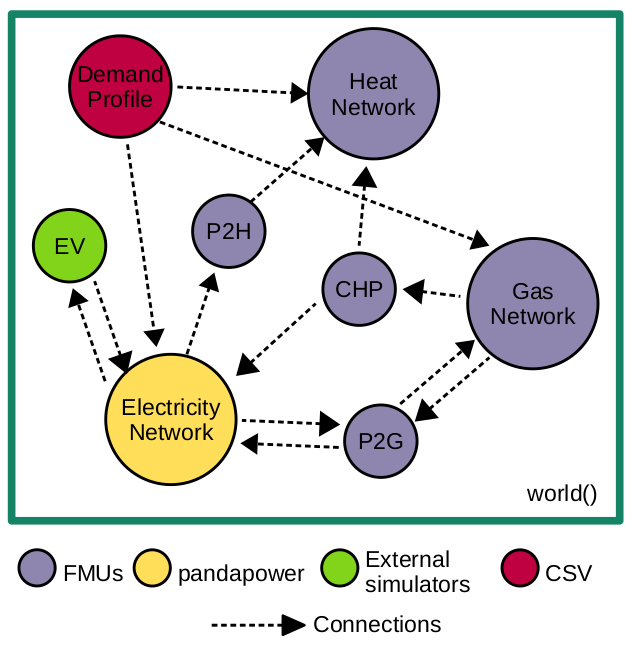# energysim documentation¶

Compatible with Python 3.6 and above.

## What is energysim?¶

`energysim` is a python based cosimulation tool designed to simplify multi-energy cosimulations. The tool was initially called `FMUWorld`, since it focussed exclusively on combining models developed and packaged as Functional Mockup Units (FMUs). However, it has since been majorly updated to become a more generalisable cosimulation tool to include a more variety of energy system simulators.

The idea behind development of `energysim` is to simplify cosimulation to focus on the high-level applications, such as energy system planning, evaluation of control strategies, etc., rather than low-level cosimulation tasks such as message exchange, time progression coordination, etc.

Currently, `energysim` allows users to combine:

1. Dynamic models packaged as Functional Mockup Units.
2. Pandapower networks packaged as pickle files.
3. PyPSA models (still under testing) as Excel files.
4. User-defined external simulators interfaced with .py functions.
5. CSV data files## Installation¶

`energysim` can be installed with `pip` using:

```pip install energysim
```

### Dependencies¶

`energysim` requires the following packages to work:

1. FMPy
2. Pandapower
3. PyPSA
4. NumPy
5. Pandas
6. Matplotlib
7. NetworkX
8. tqdm

## Usage¶

`energysim` cosimulation is designed for an easy-plug-and-play approach. The main component is the `world()` object. This is the “playground” where all simulators, and connections are added and the options for simulation are specified. `world()` can be imported by implementing:

```from energysim import world
```

### Initialization¶

Once `world` is imported, it can be initialized with basic simulation parameters using:

```my_world = world(start_time=0, stop_time=1000, logging=True, t_macro=60)
```

`world` accepts the following parameters :

• `start_time` : simulation start time (0 by default).
• `stop_time` : simulation end time (1000 by default).
• `logging` : Flag to toggle update on simulation progress (True by default).
• `t_macro` : Time steps at which information between simulators needs to be exchanged. (60 by default).

After initializing the world cosimulation object, simulators can be added to the world using the `add_simulator()` method:

```my_world.add_simulator(sim_type='fmu', sim_name='FMU1',
sim_loc=/path/to/sim, inputs=['v1', 'v2'], outputs=['var1','var2'], step_size=1)
```

where:

• `sim_type` : ‘fmu’, ‘powerflow’, ‘csv’, ‘external’
• `sim_name` : Unique simulator name.
• `sim_loc` : A raw string address of simulator location.
• `outputs` : Variables that need to be recorded from the simulator during simulation.
• `inputs` : Input variables to the simulator.
• `step_size` : Internal step size for simulator (1e-3 by default).

Please see documentation on `add_simulator` to properly add simulators to `energysim`. The values to simulator input are kept constant for the duration between two macro time steps.

### Connections between simulators¶

Once all the required simulators are added, the connections between them can be specified with a dictionary as follows

```connections = {'sim1.output_variable1' : 'sim2.input_variable1',
'sim3.output_variable2' : 'sim4.input_variable2',
'sim1.output_variable3' : 'sim2.input_variable3',}
```

This dictionary can be passed onto the world object:

```my_world.add_connections(connections)
```

### Initializing simulator variables¶

Initialization is important to start-up simulator in a cosimulation. If the simulators are not internally initialized, or of users want to use different initial conditions for the simulators, it can easily be done in `energysim`. To provide initial values to the simulators, an `init` dictionary can be specified and given to the `world` object

```initializations = {'sim_name1' : (['sim_variables'], [values]),
'sim_name2' : (['sim_variables'], [values])}
options = {'init' : initializations}
my_world.options(options)
```

### Executing simulation¶

Finally, the `simulate()` function can be called to simulate the world. This returns a dictionary with simulator name as keys and the results of the simulator as pandas dataframe. `pbar` can be used to toggle the progress bar for the simulation:

```my_world.simulate(pbar=True)
```

### Extracting Results¶

Results can be extracted by calling `results()` function on `my_world` object. Additionally, `to_csv` flag can be toggled to export results to csv files. If `False`, the function returns a dictionary object with each simulators’ results as pandas dataframe:

```results = my_world.results(to_csv=True)
```